# IRP Classes: Send and receive infrared commands via serial port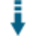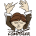Download .zip
 InfoExampleView files (16)Download .zip Reputation Support forum Blog Links
 Ratings Unique User Downloads Download Rankings Not yet rated by the users Total: 102 All time: 9,707 This week: 106Version License PHP version Categories irpclasses 1.04 GNU Lesser Genera... 5 PHP 5, Wireless and Mobile, Hardware
Description

Author

This class can send and receive infrared commands via serial port.

It can parse IRP strings that define communication sequences between infrared devices.

The class can encode and decode command sequences to be sent and received from remote controls.

Innovation AwardAugust 2017 Number 9 Infrared radiation is used to transmit information between remote control devices and other devices controlled remotely. This class can send commands to infrared devices using sequences defined using the IRP notation format. Manuel LemosPerformance Level
Name: Classes: Marco Sillano `` 4 packages by Marco Sillano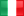Italy ??? 2019 79 in Italy362 in ItalyInnovation awardNominee: 3x

Winner: 1x

## Example

 ``` ?(16,-8,(D:8,F:8,1,-45)+)'; \$JVC_data = '{D=7,F=0x4F}'; // alternative: here as example, not used: \$JVC_HEX = 'E0FC'; // alternative: here as example, not used: \$data_JVC = array(); \$data_JVC['D'] = 7; \$data_JVC['F'] = 0x3F; \$Denon = '{38k,264}<1,-3|1,-7>(D:5,F:8,0:2,1,-165,D:5,~F:8,3:2,1,-165)+'; \$Denon_data = '{D=7,F=0x3F}'; \$NEC2 = '{38.0k,564}<1,-1|1,-3>(16,-8,D:8,S:8,F:8,~F:8,1,^108m)+'; \$NEC2_data = '{D=0,S=191,F=1}'; \$NEC1 = '{38.0k,562}<1,-1|1,-3>(16,-8,D:8,~D:8,F:8,~F:8,1,^110m,(16,-4,1,^110m)*)'; //\$NEC1='{38.0k,564}<1,-1|1,-3>(16,-8,D:8,S:8,F:8,~F:8,1,^108m,(16,-4,1,^108m)*)'; \$NEC1_data = '{D=6,F=1}'; \$AdNotam = '{35.7K,895,msb}<1,-1|1,-3>(0:1,1:1,D:6,F:6,1,^114m)+'; \$AdNotam_data = '{D=0x17,F=0x15}'; \$Amino = '{36.0k,268,msb}<-1,1|1,-1>([T=1] [T=0],7,-6,3,D:4,1:1,T:1,1:2,0:8,F:8,15:4,C:4,-79m)+{C =(D:4+4*T+9+F:4+F:4:4+15)&15}'; \$Amino_data = '{D=7,F=0xF0}'; \$Archer = '{0k,12}<1,-3.3m|1,-4.7m>(F:5,1,-9.7m)+'; \$Archer_data = '{F=17}'; \$DirectTV = '{38k,600,msb}<1,-1|1,-2|2,-1|2,-2>(5,(5,-2,D:4,F:8,C:4,1,-50)+) {C=7*(F:2:6)+5*(F:2:4)+3*(F:2:2)+(F:2)}'; \$DirectTV_data = '{D=0x0A, F=37}'; \$Grunding16 = '{35.7k,578,msb}<-4,2|-3,1,-1,1|-2,1,-2,1|-1,1,-3,1> (806u,-2960u,1346u,T:1,F:8,D:7,-100)+'; \$Grunding16_data = '{T=0, D=0x3A, F=37}'; \$Nokia = '{36k,msb}<164,-276|164,-445|164,-614|164,-783>(412,-276,D:8,S:8,F:8,164,-10m)+'; \$Nokia_data = '{ D=0xDD, S=0x4A, F=37}'; \$OrtekMCE = '{38.6k,480}<1,-1|-1,1>([P=0][P=1][P=2],4,-1,D:5,P:2,F:6,C:4,-48m)+{C=3+#D+#P+#F}'; \$OrtekMCE_data = '{D=0x1A, F=37}'; \$RC5 = '{36k,msb,889}<1,-1|-1,1>(1:1,~F:1:6,T:1,D:5,F:6,^114m)+'; \$RC5_data = '{D=0x1A, F=37,T=0}'; \$XMP = '{38k,136,msb}<210u,-760u>(<0:1|0:1,-1|0:1,-2|0:1,-3|0:1,-4|0:1,-5|0:1,-6|0:1,-7|0:1,-8|0:1,-9|0:1,-10|0:1,-11|0:1,-12|0:1,-13|0:1,-14|0:1,-15>(T=0,(S:4:4,C1:4,S:4,15:4,OEM:8,D:8,210u,-13.8m,S:4:4,C2:4,T:4,S:4,F:16,210u,-80.4m,T=8)+)){C1=-(15+S+S::4+15+OEM+OEM::4+D+D::4):4,C2=-(15+S+S:4+T+F+F::4+F::8+F::12)&15}'; \$XMP_data = '{D=0x3A, S=0x33, F=0xFEDC, OEM=0xFe}'; \$Zenit = '{40k,520,msb}<1,-10|1,-1,1,-8>(S:1,<1:2|2:2>(F:D),-90m)+'; \$Zenit_data = '{S=1, F=43, D=4}'; \$Fujitsu_Aircon = '{38.4k,413}<1,-1|1,-3>(8,-4,20:8,99:8,0:8,16:8,16:8,254:8,9:8,48:8,H:8,J:8, K:8, L:8, M:8,N:8,32:8,Z:8,1,-104.3m)+ {H=16*A + wOn, J=16*C + B, K=16*E:4 + D:4, L=tOff:8, M=tOff:3:8+fOff*8+16*tOn:4, N=tOn:7:8+128*fOn,Z=256-(H+J+K+L+M+N+80)%256}'; // [A:0..15,wOn:0..1,B:0..15, C:0..15,D:0..15,E:0..15,tOff:0..1024,tOn:0..1024,fOff:0..1,fOn:0..1] \$Fujitsu_Aircon_data = '{A=0,wOn=1,B=2, C=3,D=4,E=5,tOff=0x10,tOn=0x20,fOff=0,fOn=0}'; // NOTA: We can add to existing expressions, calculating H,J,K,L,M,N e Z from A,wOn,B,C,D,E,tOff,tOn,fOff,fOn, // also the inverses expressions, calculating A,wOn,B,C,D,E,tOff,tOn,fOff,fOn from H,J,K,L,M,N,Z. // This will not influence the ENCODE phase (values have precedence on expressions) but in DECODE phase we can get // from RAW not only H,J,K,L,M,N,Z but also A,wOn,B,C,D,E,tOff,tOn,fOff,fOn. (using dataVerify() function) \$Fujitsu_Aircon_modified = '{38.4k,413}<1,-1|1,-3>(8,-4,20:8,99:8,0:8,16:8,16:8,254:8,9:8,48:8,H:8,J:8, K:8, L:8, M:8,N:8,32:8,Z:8,1,-104.3m)+ {H=16*A + wOn, J=16*C + B, K=16*E:4 + D:4, L=tOff:8, M=tOff:3:8+fOff*8+16*tOn:4, N=tOn:7:4+128*fOn,Z=256-(H+J+K+L+M+N+80)%256, A=H:4:4,wOn=H:1,B=J:4,C=J:4:4,D=K:4,E=K:4:4,tOff=L+256*M:3, tOn=M:4:4+16*N:7,fOn=N:1:7,fOff=M:1:3}'; \$Fujitsu_Aircon_modified_data = '{A=0,wOn=1,B=2, C=3,D=4,E=5,tOff=0x10,tOn=0x20,fOff=0,fOn=0}'; // Only this requires Data persistence ( T is not defined)... // set \$number > 1 (more commands), and call resetData() before re-run to start clean. \$test1 = '{36k,msb,889}<1,-1|-1,1>(1:1,~F:1:6,T:1,D:5,F:6,^100m,T=T+1)+'; \$test1_data = '{D=0x0D, F=0x7F}'; // ====================================== test code ========== echo ''; echo " ==== ENCODING/DECODING RAW IR PROTOCOL \$protocol ====

"; echo " ==== PROTOCOL INFOS by toString() ====
"; // create object \$aProtocol = new irp_protocol(\$\$protocol); if (\$protocol == 'test1')     \$aProtocol->setDataPermanence(); // 'test1' protocol requires data permanence // prints some infos about protocol \$aProtocol->toString(); // data name \$data = \$protocol . '_data'; echo '
==== ENCODE: RAW output from encodeRaw():
'; \$raw1 = \$aProtocol->encodeRaw(\$\$data, \$number); // RAW is default print('RAW = ' . \$raw1 . '
'); echo '
==== ENCODE: RAW output compressed by RAWprocess():
'; \$raw2 = \$aProtocol->RAWprocess(\$raw1, 0); print('RAW-0 = ' . \$raw2 . '
'); print('RAW-1 = ' . \$aProtocol->RAWprocess(\$raw1, 1) . '
'); print('RAW-2 = ' . \$aProtocol->RAWprocess(\$raw1, 2) . '
'); echo '
==== ENCODE: user data
'; echo 'DATA = ' . \$\$data . '
'; echo '
==== DECODE: RAW output by decodeRaw(), using RAW_0 as input:
'; \$aProtocol->resetData(); // in case of permanence, restart \$out = \$aProtocol->decodeRaw(\$raw2); print('DATA = ' . \$out . '
'); echo '
==== DECODE: dataVerify(false) - terse output:
'; echo '
' . \$aProtocol->dataVerify(false) . '
'; echo '
==== DECODE: dataVerify(true) - verbose output:
'; echo '
' . \$aProtocol->dataVerify(true) . '
'; echo '
==== COMPARISON ENCODE/DECODE BIN
'; // now output mode BIN: \$aProtocol->setOutputBin(); // get DECODE again with output bin \$aProtocol->resetData(); // in case of permanence, restart \$bin2 = \$aProtocol->decodeRaw(\$raw2); // get ENCODE again with output bin \$aProtocol->resetData(); // in case of permanence, restart \$bin = \$aProtocol->encodeRaw(\$\$data, \$number); print('
E-BIN   = ' . \$bin . '
'); print('D-BIN = ' . \$bin2 . '
'); // transform BINs using RAWprocess() print('E-BIN-0 = ' . \$aProtocol->RAWprocess(\$bin, 0) . '
'); print('D-BIN-0 = ' . \$aProtocol->RAWprocess(\$bin2, 0) . '
'); print('E-BIN-1 = ' . \$aProtocol->RAWprocess(\$bin, 1) . '
'); print('D-BIN-1 = ' . \$aProtocol->RAWprocess(\$bin2, 1) . '
'); print('E-BIN-2 = ' . \$aProtocol->RAWprocess(\$bin, 2) . '
'); print('D-BIN-2 = ' . \$aProtocol->RAWprocess(\$bin2, 2) . '

'); echo '
<<< Back
'; echo ''; ?> ```

# irp_classes

This php classe implements the core algorithms required for working with IR remote controls, i.e. encode and decode RAW IR commands, using informations about the IR protocol in 'IRP notation'. The irp_classes is an 'execution process' for IRPs, extended to encode and decode processes of IR raw streams.

In this library you also found some methods to handle RAW data streams, without IRP, for 'learn and repeat' applications. irp_classes was designed to help me (and you) to build good applications using IR Remote Control, like media home automation, with known IRPs and not for analysing or reverse-engineering unknown IR protocols, because for that are many better applications (IRremote, IrScrutinizer etc..).

The global desing is different from usual: Arduino (or other hardware) does only very simple tasks: to receive and to transmit RAW IR signals. All the work is done on PHP, and it is universal, based on IRPs, no size or protocols limits. The irp_class can be used with a big database in WAMP environment, as I do in remotesDB (https://github.com/msillano/remotesDB), library and demo application for replica of any IR remote control (air condioners included).

To store and retrieve IR commands you have many options: - RAW: big size but fast, car it not requires IRP or processing (format like RAW-0 or any compressed version: RAW-1,RAW-2) - HEX: small, but requires an IRP (format like BIN-1 or BIN-2) - DATA SET: smallest and significant (like {D=7,F=0x3F})

With this library you have also 2 test pages: 1. Test cases for the library irp_classes: encode + decode. This test don't requires hardware. It produces a RAW IR stream, then decodes it. See examples/example_Fujitsu_aircon_modified_test.pdf. 2. (receive) + decode and analyse test. This demo can run with capturing HW (Arduino) or it can use some recorded IR RAW data. See examples/example_NEC1_decode.pdf.

Arduino: the HW schema and the Arduino sketch can be found in the dir 'Arduino'

LIMITS - Raw stream receive precision = +/- 1 microsecond (with Arduino uno Rev.3: +/- 2 microseconds) - Raw transmit frequencies: no limits (with Arduino uno Rev.3: 30KHz - 57 KHz) - Raw receive buffer size: no limits (with Arduino uno Rev.3: 300) - Raw transmit size:no limits (with Arduino uno Rev.3: 300)

WORK IN PROGRESS project, now composed of following modules:

1) irp_classes (https://github.com/msillano/irp_classes): base classes and libray to work with IRP and RAW IR streams. Demo: (receive) + decode and analyse IR commands.

2) remotesDB (https://github.com/msillano/remotesDB): MySQL DB for IRP applications and related php tools. Demo: replica of any remote control in DB.

3) remotesDBdiscovery (https://github.com/msillano/remotesDB): This extension of remotesDB is for the management of new remote controls, with 100+ IRP

4) USBphpTunnel_fifo (https://github.com/msillano/USBphpTunnel_fifo): This Android app allows you to use a TVbox (e.g. MXQ) as stand alone web server running remotesDB, talking via USB with an Arduino board as IR interface.

INSTALLATION

Copy all in web area of your WAMP server: e.g. ' ...\apache\htdocs\www\phpIRPlib'.

optional: If you have Arduino-uno and an IR receiver:

`````` -  See the dir Arduino
``````

optional: Serial communications php-Arduino in windows:

``````-  Download and install 'PHP Serial extension' free from http://www.thebyteworks.com (with some limits).
``````

note: If you have some different IR HW, modify irp_rxtxArduino.php to receive RAW data from your HW.Files
File Role DescriptionArduino (3 files)css (1 file)examples (4 files)decode-test.php Example Example scriptfull-test.php Example Example script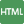index.html Doc. Documentationirp_classes.php Class Class sourceirp_rxtxArduino.php Aux. Auxiliary scriptLICENSE Lic. License textREADME.md Doc. Documentationreadme.txt Doc. DocumentationFiles / Arduino
File Role Description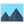arduinoIR.jpg Data Auxiliary dataIRHW-Arduino.pdf Data Auxiliary datarawRxTx02.ino Data Auxiliary dataFiles / css
File Role Descriptionstyle.css Data Auxiliary dataFiles / examples
File Role Descriptiondemo_indexpage.gif Data Auxiliary datademo_indexpage.pdf Data Auxiliary dataexample_Fujitsu_aircon_modified_test.pdf Data Auxiliary dataexample_NEC1_decode.pdf Data Auxiliary data

 All time: 9,707 This week: 106About usAdvertise on this siteSite mapNewsletterStatisticsSite tipsPrivacy policyContact
For more information send a message to `info at phpclasses dot org`.